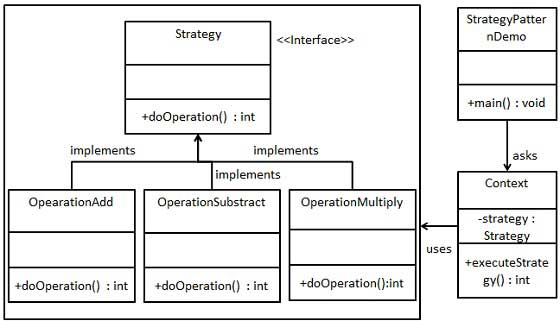# Design Patterns - Strategy Pattern

In Strategy pattern, a class behavior or its algorithm can be changed at run time. This type of design pattern comes under behavior pattern.

In Strategy pattern, we create objects which represent various strategies and a context object whose behavior varies as per its strategy object. The strategy object changes the executing algorithm of the context object.

## Implementation

We are going to create a Strategy interface defining an action and concrete strategy classes implementing the Strategy interface. Context is a class which uses a Strategy.

StrategyPatternDemo, our demo class, will use Context and strategy objects to demonstrate change in Context behaviour based on strategy it deploys or uses.## Step 1

Create an interface.

Strategy.java

```public interface Strategy {
public int doOperation(int num1, int num2);
}
```

## Step 2

Create concrete classes implementing the same interface.

```public class OperationAdd implements Strategy{
@Override
public int doOperation(int num1, int num2) {
return num1 + num2;
}
}
```

OperationSubstract.java

```public class OperationSubstract implements Strategy{
@Override
public int doOperation(int num1, int num2) {
return num1 - num2;
}
}
```

OperationMultiply.java

```public class OperationMultiply implements Strategy{
@Override
public int doOperation(int num1, int num2) {
return num1 * num2;
}
}
```

## Step 3

Create Context Class.

Context.java

```public class Context {
private Strategy strategy;

public Context(Strategy strategy){
this.strategy = strategy;
}

public int executeStrategy(int num1, int num2){
return strategy.doOperation(num1, num2);
}
}
```

## Step 4

Use the Context to see change in behaviour when it changes its Strategy.

StrategyPatternDemo.java

```public class StrategyPatternDemo {
public static void main(String[] args) {
Context context = new Context(new OperationAdd());
System.out.println("10 + 5 = " + context.executeStrategy(10, 5));

context = new Context(new OperationSubstract());
System.out.println("10 - 5 = " + context.executeStrategy(10, 5));

context = new Context(new OperationMultiply());
System.out.println("10 * 5 = " + context.executeStrategy(10, 5));
}
}
```

## Step 5

Verify the output.

```10 + 5 = 15
10 - 5 = 5
10 * 5 = 50
```